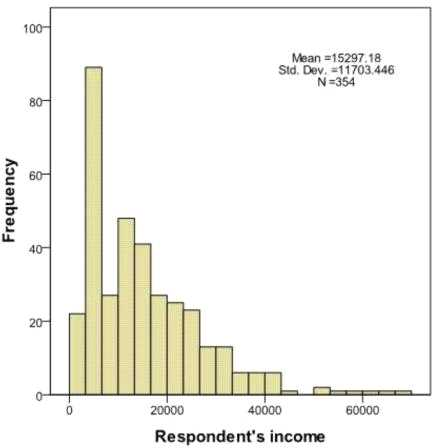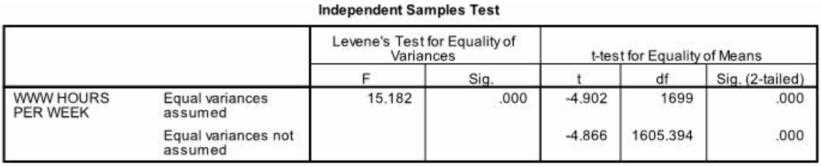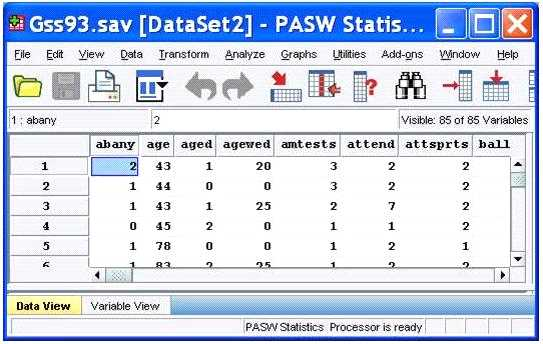# IBM c2090-011 practice test

## IBM SPSS Statistics Level 1 v2 Exam

##### Question 1

Which chart type is appropriate for viewing the association between two scale variables?

• A. Bar chart
• B. Area chart
• C. Pie chart
• D. Scatter plot

D

Discussions
0 / 600

##### Question 2

The reason you would select the check box next to indicate case source variable in the
Add Cases dialog box is to:

• A. Create a new case in your merged data.
• B. Highlight a specific case in one of your variables.
• C. Create a variable identifying the case data source.
• D. Create a variable identifying the variable data source.

C

Discussions
0 / 600

##### Question 3

You have been asked to create a categorical variable from a scale variable, income, illustrated
in the histogram below. The resulting categorical variable must have 5 categories with an
approximately equal number of cases in each category. Which way would you accomplish
this using the IBM SPSS Statistics Visual Binning dialog?• A. Use the automatic option Equal Width Intervals
• B. Use the automatic option Equal Percentiles Based on Scanned Cases
• C. Use the automatic option Outpoints at Mean and Selected Standard Deviations Based on Scanned Cases
• D. Specify Cut points manually at 10000, 20000, 30000, 40000 & 50000.

B

Discussions
0 / 600

##### Question 4

Which interpretation is correct for the Independent Samples T-Test table shown below?• A. We did not meet the assumption of homogeneity of variance; however our two groups differed on the number of WWW HOURS PER WEEK.
• B. We did meet the assumption of homogeneity of variance and our two groups differed on the number of WWW HOURS PER WEEK.
• C. We did not meet the assumption of homogeneity of variance and our two groups did not differ on the number of WWW HOURS PER WEEK. We did meet the assumption of homogeneity of variance; however our two groups did not differ on the number of WWW HOURS PER WEEK.

A

Discussions
0 / 600

##### Question 5

As the sample size increases, the amount of variabilit9 in the distribution of sample means
increases.

• A. True
• B. False

B

Discussions
0 / 600

##### Question 6

The IBM SPSS Statistics Select Cases provides various ways to select cases included in
further analysis. If you needed to conduct analysis on those respondents whose age is more
than 30 years, earning in excess of \$40000, which option in this dialog would you choose to
specify these criteria?

• A. Random sample of cases
• B. Based on time or case range
• C. Use filter variable
• D. If condition is satisfied

D

Discussions
0 / 600

##### Question 7

In the Aggregate data procedure1 if you specify more than one Break Variable, ________ in
the Break Variables list will determine case order.

• A. Variable size
• B. Variable label
• C. Variable name
• D. Variable order

D

Discussions
0 / 600

##### Question 8

There are two major types of errors one can make when attempting to draw conclusions
about the larger population based on the information collected in the sample. For example,
when performing an Independent Samples T-Test, one type of error that can occur is when
there are significant differences between the groups based on a scale level dependent
variable, but the test fails to detect them. Which of the following choices describe this sort of
error? (Choose two.)

• A. Type i error
• B. Type ii error
• C. False negative
• D. False positive

B, C

Discussions
0 / 600

##### Question 9

With IBM SPSS Statistics you can assign one of three levels of measurement to a variable.
Which is the correct list of all three levels?

• A. Nominal - Ordinal - Interval
• B. Categorical - Interval - Ratio
• C. Nominal - Ordinal - Scale
• D. Nominal - Interval – Scale

C

Discussions
0 / 600

##### Question 10

After studying the figure below1 select the correct statement.• A. Gss93.sav is the dataset name.
• B. DataSet2 is the dataset name.
• C. DataSet2 is the file name.
• D. DataSet2 is both the file and dataset name.